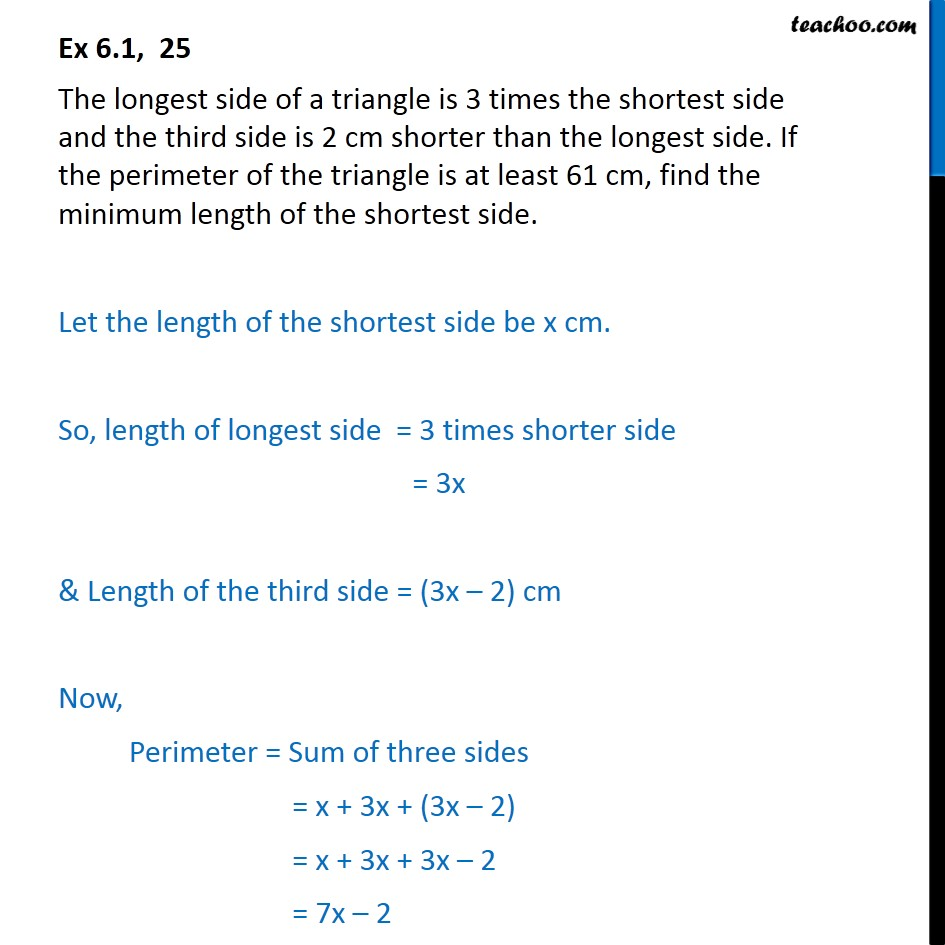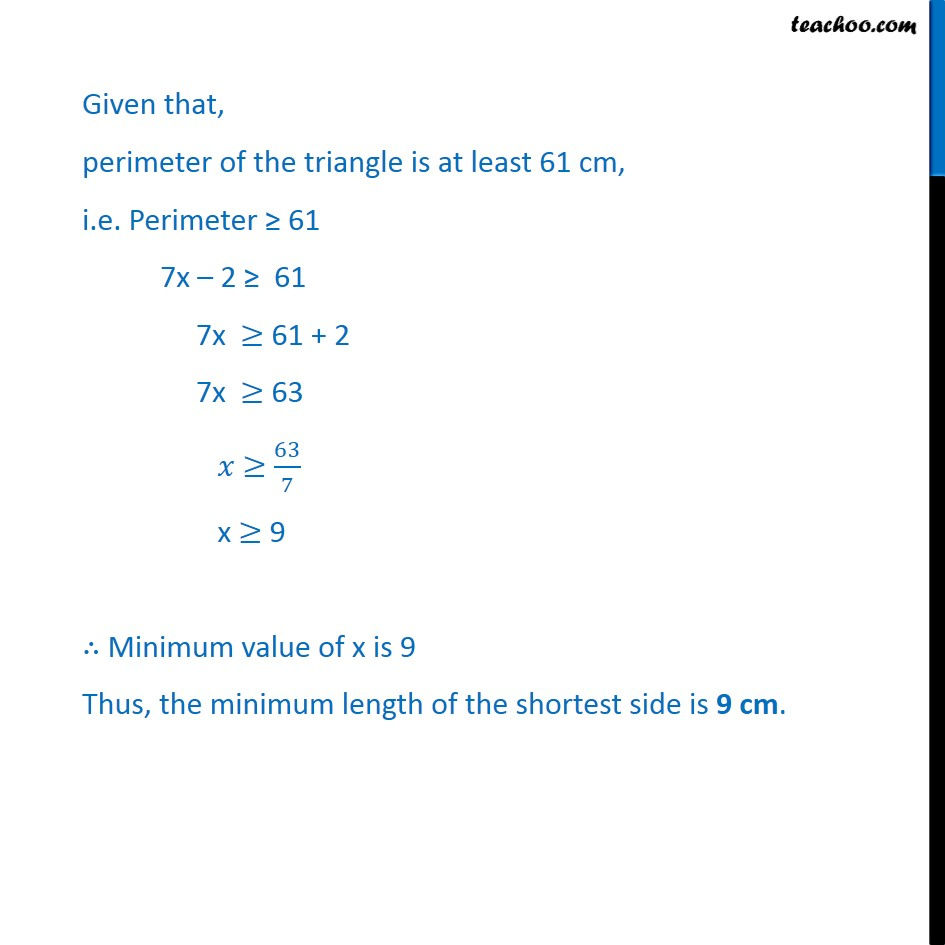1. Class 11
2. Important Question for exams Class 11
3. Chapter 6 Class 11 Linear Inequalities

Transcript

Ex 6.1, 25 The longest side of a triangle is 3 times the shortest side and the third side is 2 cm shorter than the longest side. If the perimeter of the triangle is at least 61 cm, find the minimum length of the shortest side. Let the length of the shortest side be x cm. So, length of longest side = 3 times shorter side = 3x & Length of the third side = (3x – 2) cm Now, Perimeter = Sum of three sides = x + 3x + (3x – 2) = x + 3x + 3x – 2 = 7x – 2 Given that, perimeter of the triangle is at least 61 cm, i.e. Perimeter ≥ 61 7x – 2 ≥ 61 7x ≥ 61 + 2 7x ≥ 63 𝑥 ≥ ﷐63﷮7﷯ x ≥ 9 ∴ Minimum value of x is 9 Thus, the minimum length of the shortest side is 9 cm.

Chapter 6 Class 11 Linear Inequalities

Class 11
Important Question for exams Class 11